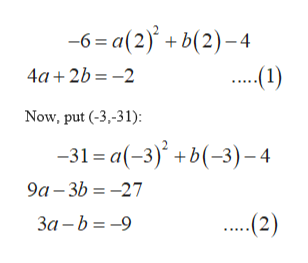# For Exercise, find an equation of the form  y = ax2 + bx + c that defines the parabola through the three noncollinear points given.(0, −4), (2, −6), (−3, −31)

Question
2 views

For Exercise, find an equation of the form  y = ax2 + bx + c that defines the parabola through the three noncollinear points given.

(0, −4), (2, −6), (−3, −31)

check_circle

Step 1

Consider the given equation of parabola:

Step 2

Now, parabola passes through the point (0, - 4), so it will satisfy the equation:

Step 3

Now, put the remaining points in the equation y=ax...help_outlineImage Transcriptionclose-6 = a(2)' + b(2)–4 .(1) 4a + 2b = -2 Now, put (-3,-31): -31 = a(-3)' +b(-3)– 4 9a – 3b = -27 За -b %3D —9 (2) fullscreen

### Want to see the full answer?

See Solution

#### Want to see this answer and more?

Solutions are written by subject experts who are available 24/7. Questions are typically answered within 1 hour.*

See Solution
*Response times may vary by subject and question.
Tagged in

### Other